# MP Board Class 9th Maths Solutions Chapter 11 Constructions Ex 11.1

## MP Board Class 9th Maths Solutions Chapter 11 Constructions Ex 11.1

Question 1.
Construct an angle of 90° at the initial point of a given ray and justify the construction.
Solution:1. Draw a ray OA and with O as centre and any radius, draw an arc, cutting OA at B.
2. With B as centre and the same radius draw an arc cutting the arc drawn in step (i) at C.
3. With C as centre draw another arc with same radius cutting the arc drawn in step (i) at D.
4. with C as centre and the same radius draw an arc.
5. With D as centre and the same radius draw an arc, cutting the arc drawn in step (iv) at E.
6. Draw OE ∴ ∠AOF = 90°Question 2.
Construct an angle of 45° at the initial point of a given ray justify the construction.
Solution:

1. Draw ∠AOF = 90° by following the same steps for constructing a 90° angle.
2. Draw OG, the bisector of ∠AOF.
3. ∠AOF= 45°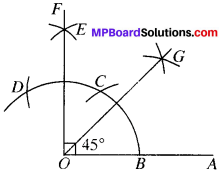Question 3.
Construct the angle of the following measurements:
Solution:

1. 30°
2. 22 $$\frac{1}{2}$$°
3. 15°

1. 30°

1. Draw a ray OA.
2. With O as centre and any radius draw an arc which intersect OA at B.
3. With B as centre and same radius draw an arc cutting the arc drawn is step (ii) at C.
4. Join OC and produce upward
5. ∠BOC = 60°
6. Draw the bisector OD of ABOC.
7. ∠BOD = ∠COD = 30°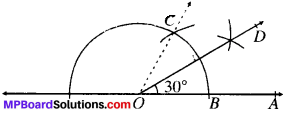2. 22 $$\frac{1}{2}$$°

1. Draw a ray OA.
2. With O as centre and any radius draw an arc which intersect intersect CM at B.
3. With B as centre and same radius draw an arc intersecting the arc drawn in step (ii) at C.
4. With C as centre and same radius draw an arc cutting the arc drawn in step (ii) at D.5. With C and D as centres and same radius draw arcs to intersect at E.
6. Join OE.
7. ∠AOG – 90°
8. Draw the bisector OE of ∠AOE, to get ∠AOF = 45°
9. Draw the bisector OG of ∠AOF.
10. ∠AOG = 22$$\frac{1}{2}$$°

3. 15°

1. Draw a ray CM.
2. With O as centre and any radius draw an arc which intersect CM at B.
3. With B as centre and same radius draw an arc intersecting the arc drawn in step (ii), at C.
4. Draw OD as the bisector of ZAOC.
5. ∠BOD = 30°
6. Draw the bisector OE of ∠AOD.
7. ∠AOE = 15°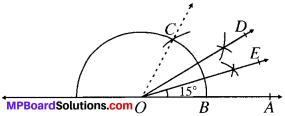Question 4.
Construct the following angles and verily by measuring them by a protractor.

(i) 75°
(ii) 105°
(iii) 135°

Solution:Question 5.
Construct an equilateral triangle given its side and justify the construction.
Solution:

1. Draw a ray AY with intial point A.
2. With centred and radius equal to length of a side of the A draw an arc BY, cutting the ray AX at B.
3. With centre B and the same radius draw an arc cutting are BY at C.
4. Join AC and BC to obtain the required A.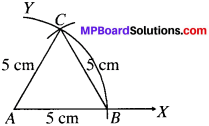Construction of Triangles:

Example 1:
Construction of a Triangle when its base, a base angle and sum of other two sides are given
Solution:
Step of Construction:

1. Draw the base PQ.
2. At point P draw an angle, MPQ equal to the given angle.
3. Cut a line segment PM equal to sum of sides i.e., (PR + RQ) from point P.
4. Join MQ.
5. Draw the perpendicular bisector of MQ which intersect PM at R.
6. Join QR. PQR is the required triangle.Justification:
Mark points S as shown in Fig.
QS = MS (∴ RS is the perpendicular bisector of MQ)
RS = RS (Common)
∠QSR = ∠MSR (Each $${ 90 }^{ \underline { 0 } }$$)
∆RSQ ≅ ∆RSQ (By SAS)
and so PQ = RM (By CPCT)
Now PR = PM – RM = PM – RQ
PM = PR + RQ.Example 2:
Construct a AABC in which BC = 3.6 cm, AB + AC = 4.8 and A RSM QS – MS RS = RS cm and B = ∠60°.
Solution:
Steps of Construction:

1. Draw BC = 3.6 cm.
2. Draw ∠CBX= $${ 60 }^{ \underline { 0 } }$$ at B.
3. From BX, cut off line segment BD = 4.8 cm.
4. Join DC.
5. Draw the perpcndicub: bisector ofDC meeting BD at A.
6. joinAC.
7. ABC is the required Mangle.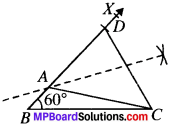Justification:
‘A‘ lies on the perpendicular bisector of DC
Now BD = 4.8 cm
⇒ BA + AD = 4.8
BA + AC = 4.8 (∴ AD = AC)

Example 3:
Construction of a Triangle when its Base Angle and the Difference of the other two sides are given.
Solution:
Case I:
Given the base BC, a base angle, say ∠B and AB – AC. Steps of Construction:

1. Draw the base BC.
2. At point B draw an angle ∠CBX equal to the given angle.
3. Cut a line segment BD = AB – AC from point B.
4. Join DC.
5. Draw the perpendicular bisector of . DC which intersect BX at A.
6. Join AC.
7. ΔABC is the required triangle.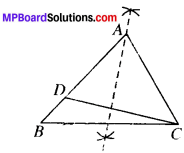Justification:
As point A lies on the perpendicular bisector of DC
BD = AB – AD = AB – AC (∴ AD = AC)

Case II:
Given the base BC, a base angle say ∠B and (AC – AB).
Steps of Construction:

1. Draw the base SC.
2. At point S, draw an angle, ∠CBX equal to the given angle and extend the arm XB backward.
3. Cut a line segment BD equal to (AC – AB) from the extended arm.
4. Join DC.
5. Draw the perpendicular bisector of DC which intersect BX at A.
6. Join AC.
7. ΔABC is the required triangle.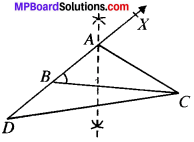Justification:
As the perpendicular bisector of DC passes through A.
∴ BD = AC – AB

Example 4:
Construct a ∆ABC in which BC = 3.4 cm, AB – AC = 1.5 cm and ∠B = $${ 45 }^{ \underline { 0 } }$$.
Solution:
Steps of Construction:

1. Draw base BC = 3.4 cm.
2. Draw ∠CBX = $${ 45 }^{ \underline { 0 } }$$.
3. From BX, cut line segment BD =1.5 cm.
4. Join DC.
5. Draw the perpendicular bisector of DC which intersect BX at A.
6. Join AC.
7. ABC is the required triangle.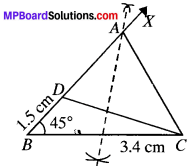Justification:
As A lies on perpendicular bisector of DC.
BD = 1.5 cm
⇒ AB – AD =1.5 cm
AB – AC =1.5 cmExample 5:
Construct a ∆PQR in which QR = 5.8 cm, PR – PQ = 1.8 cm and ∠Q = $${ 45 }^{ \underline { 0 } }$$. Justify your construction.
Solution:
Steps of Construction:

1. Draw base QR = 5.8 cm.
2. Draw ∠RQP = $${ 45 }^{ \underline { 0 } }$$.
3. Produce arm XQ backward and cut a line segment QS = 1.8 cm.
4. Join SR.
5. bisector of SR which intersect QX at P.
6. Join PR.
7. PQR is the required triangle.Justification:
As P lies on perpendicular bisector of SR
PS = PR
QS = 1.8 cm
⇒ PS – PQ = 1.8 cm
∴ PR – PQ = 1.8 cm

Example 6:
Construct a ∆ABC in which BC = 5.8 cm, ∠C = $${ 60 }^{ \underline { 0 } }$$ and AB – AC = 2.5 cm.
Solution:
Steps of Construction:

1. Draw base BC = 5.8 cm
2. Draw ∠ACB = $${ 60 }^{ \underline { 0 } }$$
3. Produce arm CX backward and cut a line segment CD = 2.5 cm.
4. Join BD.
5. Draw perpendicular bisector of BD which intersect CX at A.
6. Join AB.
7. ABC is the required triangle.Justification:
A lies on the perpendicular bisector of BD.
CD = 2.5 cm
⇒ AD – AC = 2.5 cm
⇒ AB – AC = 2.5 cm

Example 7:
Construct a ∆ABC in which AC – AB = 3.5 cm, BC = 6.2 cm and ∠C = $${ 45 }^{ \underline { 0 } }$$.
Solution:
Steps of Construction:

1. Draw BC = 6.2 cm
2. Draw ∠ACB = $${ 45 }^{ \underline { 0 } }$$
3. Cut CD = 3.5 cm from CX.
4. Join BD and draw the perpendicular bisector of BD which intersect CX at A.
5. Join AB.
6. ABC is the required triangle.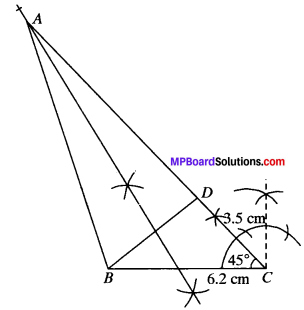Justification:
As A lies on the perpendicular bisector of BD.
CD = 3.5 cm
⇒ AC – AD = 3.5 cm
∴ AC – AB = 3.5 cm

Example 8:
Construction of a Triangle when its Perimeter and Two Base Angles are given.
Solution:
Given the base angles, ∠B and ∠C and perimeter, i.e., AB + BC + AC.
Steps of Construction:

1. Draw a line segment, DE equal to AB + BC + CA.
2. Draw ∠EDM and ∠DEN equal to the base angles ∠B and ∠C respectively.
3. Draw bisectors of ∠MDE and ∠NED which intersect at A.
4. Draw the perpendicular bisectors of AD and AE which intersect DE at B and C respectively.
5. Join AE and AC.
6. ABC is the required triangle.Justification:
As B lies on the perpendicular bisector of AD
∴ AB = DB
AB = DB
(∴ Angles opposite to equal sides of a A are equal)
Similarly, AC = CE
and ∠CAE = ∠CEA
Now DE = DB + BC + CE
= AB + BC + AC
In ∆ACE, ∠ACB = ∠CAE + ∠CEA = ∠CEA + ∠CEA = 2∠CEAExample 9:
Construct a triangle whose perimeter is 6.4 cm and angles at the base are $${ 60 }^{ \underline { 0 } }$$ and $${ 45 }^{ \underline { 0 } }$$.
Solution:
Steps of Construction:

1. Draw line segment DE equal to (AB + BC + CA) = 6.4 cm
2. Draw ∠EDM = $${ 60 }^{ \underline { 0 } }$$ and ∠DEN = $${ 45 }^{ \underline { 0 } }$$.
3. Draw AD and AE as bisectors of ∠MDE and ∠NED which inter sect at A.
4. Draw the perpendicular bisector of AD and AE which intersect DE at B and C respectively.
5. Join AB and AC.
6. ABC is the required triangle.